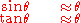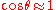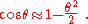xParaxial approximationEncyclopedia
In geometric optics, the paraxial approximation is a small-angle approximation used in Gaussian optics
Gaussian optics
Gaussian optics is a technique in geometrical optics that describes the behaviour of light rays in optical systems by using the paraxial approximation, in which only rays which make small angles with the optical axis of the system are considered. In this approximation, trigonometric functions can...

and ray tracing
Ray tracing (physics)
In physics, ray tracing is a method for calculating the path of waves or particles through a system with regions of varying propagation velocity, absorption characteristics, and reflecting surfaces. Under these circumstances, wavefronts may bend, change direction, or reflect off surfaces,...

of light through an optical system (such as a lens
Lens (optics)
A lens is an optical device with perfect or approximate axial symmetry which transmits and refracts light, converging or diverging the beam. A simple lens consists of a single optical element...

).

A paraxial ray is a ray
Ray (optics)
In optics, a ray is an idealized narrow beam of light. Rays are used to model the propagation of light through an optical system, by dividing the real light field up into discrete rays that can be computationally propagated through the system by the techniques of ray tracing. This allows even very...

which makes a small angle (θ) to the optical axis
Optical axis
An optical axis is a line along which there is some degree of rotational symmetry in an optical system such as a camera lens or microscope.The optical axis is an imaginary line that defines the path along which light propagates through the system...

of the system, and lies close to the axis throughout the system. Generally, this allows three important approximations (for θ in radian
Radian is the ratio between the length of an arc and its radius. The radian is the standard unit of angular measure, used in many areas of mathematics. The unit was formerly a SI supplementary unit, but this category was abolished in 1995 and the radian is now considered a SI derived unit...

s) for calculation of the ray's path:andThe paraxial approximation is used in Gaussian optics
Gaussian optics
Gaussian optics is a technique in geometrical optics that describes the behaviour of light rays in optical systems by using the paraxial approximation, in which only rays which make small angles with the optical axis of the system are considered. In this approximation, trigonometric functions can...

and first-order raytracing. Ray transfer matrix analysis
Ray transfer matrix analysis
Ray transfer matrix analysis is a type of ray tracing technique used in the design of some optical systems, particularly lasers...

is one method that uses the approximation.

In some cases, the second-order approximation is also called "paraxial". To second order, the approximations above for sine and tangent do not change (the next term in their Taylor series
Taylor series
In mathematics, a Taylor series is a representation of a function as an infinite sum of terms that are calculated from the values of the function's derivatives at a single point....

expansion is zero), while for cosine the second order approximation isThe paraxial approximation is accurate within 0.5% for angles under about 10° but its inaccuracy grows significantly for larger angles.

For larger angles it is often necessary to distinguish between meridional rays, which lie in a plane containing the optical axis
Optical axis
An optical axis is a line along which there is some degree of rotational symmetry in an optical system such as a camera lens or microscope.The optical axis is an imaginary line that defines the path along which light propagates through the system...

, and sagittal rays, which do not.